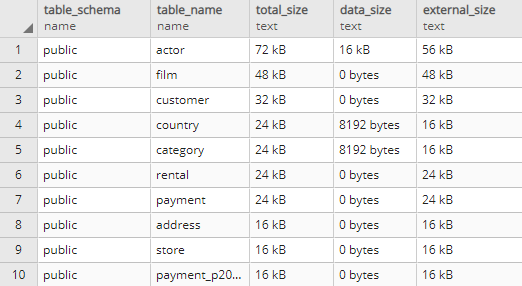## List 10 largest tables in PostgreSQL database

Article for:

This query returns list of ten largest (by data size) tables.

## Query

``````select schemaname as table_schema,
relname as table_name,
pg_size_pretty(pg_total_relation_size(relid)) as total_size,
pg_size_pretty(pg_relation_size(relid)) as data_size,
pg_size_pretty(pg_total_relation_size(relid) - pg_relation_size(relid))
as external_size
from pg_catalog.pg_statio_user_tables
order by pg_total_relation_size(relid) desc,
pg_relation_size(relid) desc
limit 10;
``````

## Columns

• table_schema - table's schema name
• table_name - table name
• total_size - total table size
• data_size - size of table's rows
• external_size - size of external elements, such as indexes etc.

## Rows

• One row represents one table
• Scope of rows: ten tables with the biggest total size
• Ordered by total, data and external size

## Sample results